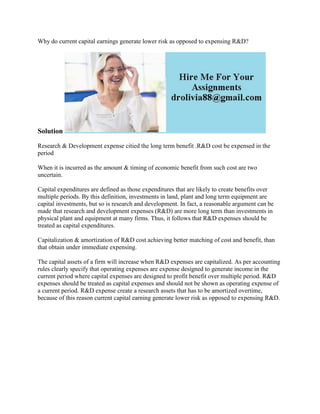Diese Präsentation wurde erfolgreich gemeldet.

# Why do current capital earnings generate lower risk as opposed to expe.docx

Anzeige
Anzeige
Anzeige
Anzeige
Anzeige
Anzeige
Anzeige
Anzeige
Anzeige
Anzeige
Anzeige×

1 von 2 Anzeige

# Why do current capital earnings generate lower risk as opposed to expe.docx

Why do current capital earnings generate lower risk as opposed to expensing R&D?
Solution
Research & Development expense citied the long term benefit .R&D cost be expensed in the period
When it is incurred as the amount & timing of economic benefit from such cost are two uncertain.
Capital expenditures are defined as those expenditures that are likely to create benefits over multiple periods. By this definition, investments in land, plant and long term equipment are capital investments, but so is research and development. In fact, a reasonable argument can be made that research and development expenses (R&D) are more long term than investments in physical plant and equipment at many firms. Thus, it follows that R&D expenses should be treated as capital expenditures.
Capitalization & amortization of R&D cost achieving better matching of cost and benefit, than that obtain under immediate expensing.
The capital assets of a firm will increase when R&D expenses are capitalized. As per accounting rules clearly specify that operating expenses are expense designed to generate income in the current period where capital expenses are designed to profit benefit over multiple period. R&D expenses should be treated as capital expenses and should not be shown as operating expense of a current period. R&D expense create a research assets that has to be amortized overtime, because of this reason current capital earning generate lower risk as opposed to expensing R&D.
.

Why do current capital earnings generate lower risk as opposed to expensing R&D?
Solution
Research & Development expense citied the long term benefit .R&D cost be expensed in the period
When it is incurred as the amount & timing of economic benefit from such cost are two uncertain.
Capital expenditures are defined as those expenditures that are likely to create benefits over multiple periods. By this definition, investments in land, plant and long term equipment are capital investments, but so is research and development. In fact, a reasonable argument can be made that research and development expenses (R&D) are more long term than investments in physical plant and equipment at many firms. Thus, it follows that R&D expenses should be treated as capital expenditures.
Capitalization & amortization of R&D cost achieving better matching of cost and benefit, than that obtain under immediate expensing.
The capital assets of a firm will increase when R&D expenses are capitalized. As per accounting rules clearly specify that operating expenses are expense designed to generate income in the current period where capital expenses are designed to profit benefit over multiple period. R&D expenses should be treated as capital expenses and should not be shown as operating expense of a current period. R&D expense create a research assets that has to be amortized overtime, because of this reason current capital earning generate lower risk as opposed to expensing R&D.
.

Anzeige
Anzeige

### Why do current capital earnings generate lower risk as opposed to expe.docx

1. 1. Why do current capital earnings generate lower risk as opposed to expensing R&D? Solution Research & Development expense citied the long term benefit .R&D cost be expensed in the period When it is incurred as the amount & timing of economic benefit from such cost are two uncertain. Capital expenditures are defined as those expenditures that are likely to create benefits over multiple periods. By this definition, investments in land, plant and long term equipment are capital investments, but so is research and development. In fact, a reasonable argument can be made that research and development expenses (R&D) are more long term than investments in physical plant and equipment at many firms. Thus, it follows that R&D expenses should be treated as capital expenditures. Capitalization & amortization of R&D cost achieving better matching of cost and benefit, than that obtain under immediate expensing. The capital assets of a firm will increase when R&D expenses are capitalized. As per accounting rules clearly specify that operating expenses are expense designed to generate income in the current period where capital expenses are designed to profit benefit over multiple period. R&D expenses should be treated as capital expenses and should not be shown as operating expense of a current period. R&D expense create a research assets that has to be amortized overtime, because of this reason current capital earning generate lower risk as opposed to expensing R&D.